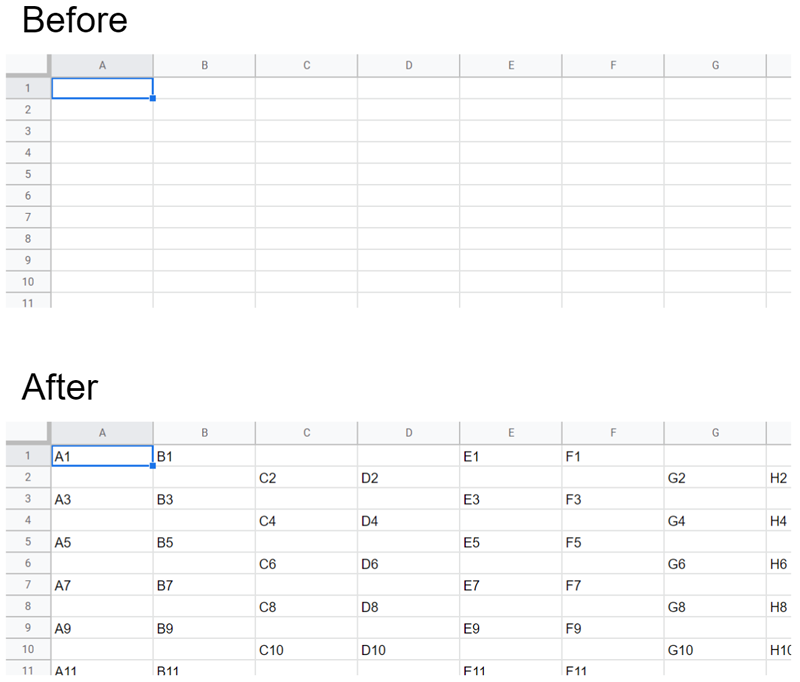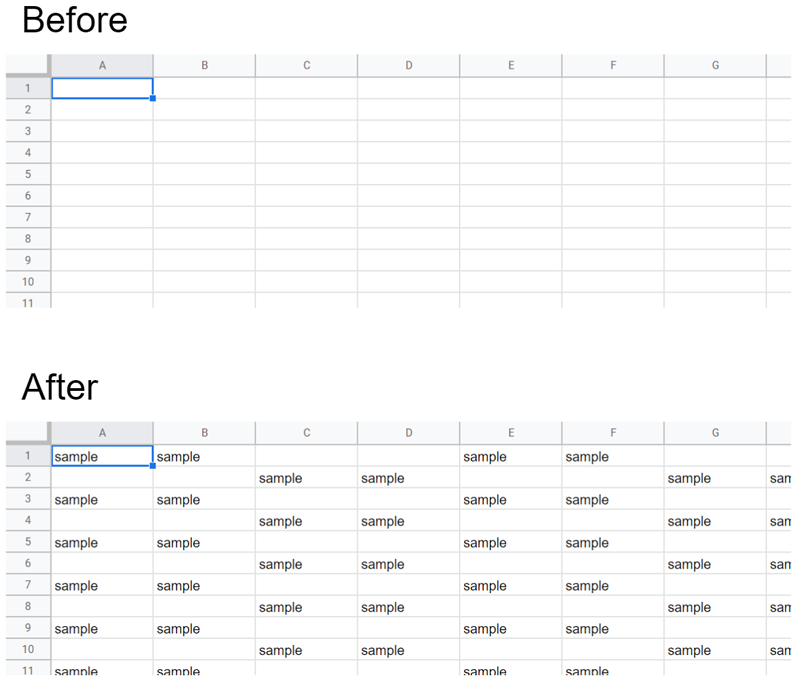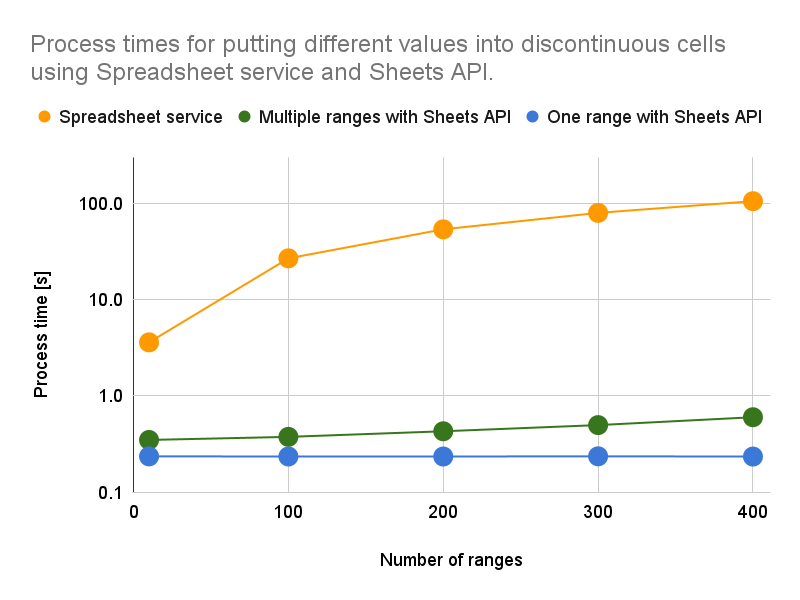Gists

## Abstract

It has already been known that Google Apps Script is a strong tool for managing Google Spreadsheets. When the values are retrieved and/or put for Google Spreadsheet, there is a case that the discontinuous cells are required to be used. This report suggests the Best Practices for processing the discontinuous cells on Google Spreadsheet. From the results of process costs, it could understand the usefulness of using the discontinuous cells with low cost using Sheets API and Class RangeList of Spreadsheet service with Google Apps Script.

## Introduction

When Google Spreadsheet is used by Google Apps Script, there is a case that the values are retrieved and/or are put for the continuous cells and the discontinuous cells. When continuous cells are used, the values can be retrieved and put for the cells from a single range using “getValues” and “setValues” methods in the Spreadsheet service as the typical methods. This has already been published as the official Best Practice of the official document. Ref

From this report, the following results were obtained.

1. When the values are retrieved from the discontinuous cells, Sheets API is suitable.

2. When the different values are put into the discontinuous cells, Sheets API is suitable.

3. When the same value is put into the discontinuous cells, the Class RangeList of Spreadsheet service is suitable.

These results could understand the usefulness of discontinuous cells using Sheets API and Class RangeList of Spreadsheet service with Google Apps Script.

In the case of the methods which are introduced in this report, not only the values but also the formulas can be used. And also, the methods using Sheets API can be also applied to the languages except for Google Apps Script.

## Sample situations and scripts

Here, 3 sample situations and scripts using the discontinuous cells are introduced.

### 1. Retrieve cell values from discontinuous cells.

In this section, it introduces the method for retrieving cell values from the discontinuous cells.

When there is a range list including A1Notation like ["A1:B1", "B2:C2", "C3:D3",,,] and you want to retrieve the values from the ranges of A1Notations, the sample script using the general method is as follows. This sample script puts each value into each range in a loop.

function sample1A() {
const ranges = ["'Sheet1'!A1:B1", "'Sheet1'!E1:F1", , ,];

const res = ranges.reduce(
(o, r) => ((o[r] = ss.getRange(r).getValues()), o),
{}
);
}


On the other hand, when Sheets API is used in this situation, the script is as follows. In this case, “Method: spreadsheets.values.batchGet” is used. When you use this script, please enable Sheets API at Advanced Google services. In the case of this script, all values from all ranges of A1Notations can be retrieved by one API call.

function sample1B() {
const ranges = ["'Sheet1'!A1:B1", "'Sheet1'!E1:F1", , ,];

ranges,
});
const res = ranges.reduce(
(o, r, i) => ((o[r] = valueRanges[i].values), o),
{}
);
}


Both sample scripts returns the following value.

{
"'Sheet1'!A1:B1":[["A1","B1"]],
"'Sheet1'!E1:F1":[["E1","F1"]],
"'Sheet1'!I1:J1":[["I1","J1"]],
,
,
,
}


### 2. Put different values into discontinuous cells.

In this section, it introduces the method for putting different values into the discontinuous cells.

When there is a range and value like [{"range":"A1:B1","values":[["A1","B1"]]},{"range":"B2:C2","values":[["B2","C2"]]},{"range":"C3:D3","values":[["C3","D3"]]},,,] and you want to put each value to each range, the sample script using the general method is as follows. This sample script puts into each value using each range in a loop.

function sample2A() {
const data = [
{ range: "'Sheet1'!A1:B1", values: [["A1", "B1"]] },
{ range: "'Sheet1'!E1:F1", values: [["E1", "F1"]] },
,
,
];

data.forEach(({ range, values }) => ss.getRange(range).setValues(values));
}


On the other hand, when Sheets API is used in this situation, the script is as follows. In this case, “Method: spreadsheets.values.batchUpdate” is used. In the case of this script, each value can be put to each range by multiple ranges with one API call. The detailed process costs are introduced in the next section of this report.

function sample2B() {
const data = [
{ range: "'Sheet1'!A1:B1", values: [["A1", "B1"]] },
{ range: "'Sheet1'!E1:F1", values: [["E1", "F1"]] },
,
,
];

{ data, valueInputOption: "USER_ENTERED" },
);
}


It introduces another approach under this situation using Sheets API as follows. The sample script can be seen at sample2C(). In the case of Sheets API, when null value is used, it is not affected by the existing cell value of the destination sheet. Ref By this, the values except for null can be put into the destination sheet by a single range with one API call.

function sample2C() {
const values = [
["A1", "B1", null, null, "E1", "F1", null, null, "I1", "J1", null, null, "M1", "N1", null, null, "Q1", "R1", null, null],
[null, null, "C2", "D2", null, null, "G2", "H2", null, null, "K2", "L2", null, null, "O2", "P2", null, null, "S2", "T2"],
,
,
];

valueInputOption: "USER_ENTERED",
});
}


As another method for values of sample2C() using the Spreadsheet service, the following flow can be also considered.

1. Retrieve values from the destination sheet using getValues.
2. Create an array by merging the existing values and new values using setValues.
3. Put the created array.

However, this process has the following problem. If the destination sheet has the values, it is required to retrieve values from the destination sheet. And, when the formulas and the rich texts are included in the destination cells, those cells are overwritten by the values without the formulas and the rich texts. If this method is used, it is required to be chosen by the situation.

When those scripts shown in this section are run, the results of all scripts are the same as shown in Fig. 1.Fig. 1. Result of spreadsheet image before and after the scripts for putting different values into discontinuous cells is run.

### 3. Put the same values into discontinuous cells.

In this section, it introduces the method for putting the same value into the discontinuous cells.

When the same values are put into the discontinuous cells, the same value is used for values of sample2A() and sample2B() in section 2. The process costs are the same. So, here, the sample script is not shown.

In the case of putting the same value to the discontinuous cells, there is a good method. That is getRangeList(a1Notations). This method has been added on April 11, 2018. And, Class RangeList has also been added. Ref When this method is used, the script can be written as follows.

function sample3A() {
const rangeList = ["A1:B1", "E1:F1", , ,];
const value = "sample";

ss.getSheetByName("Sheet1").getRangeList(rangeList).setValue(value);
}


After Class RangeList was added, this is the best way for processing not only the same values but also the same style to the discontinuous cells with the low process cost using the Spreadsheet service. For example, this can be applied for clearing the cell contents of the discontinuous cells, and also, for checking and unchecking the checkboxes of the discontinuous cells.

When this script is run, the result is as shown in Fig. 2.Fig. 2. Result of spreadsheet image before and after the scripts for putting the same value into discontinuous cells is run.

## Results and discussions

The process costs of scripts mentioned in the section “Sample situations and scripts” are shown as follows. In Figs. 3, 4, and 5, the vertical and horizontal axes are the processing time and the number of ranges, respectively. The vertical axis in all figures is the log scale.Fig. 3. Process times for retrieving cell values from discontinuous cells using Spreadsheet service and Sheets API. The results of sample1A() and sample1B() in the above sample scripts are the orange line and green line, respectively.

Figure 3 shows the process times for retrieving the cell values from the discontinuous cells using the Spreadsheet service and Sheets API. This figure indicates that when getValues of the Spreadsheet service is used in a loop, the process cost is much higher than that using Sheets API. And also, the ratio of increase in the process cost of Spreadsheet service is larger than that of Sheets API. As the specific feature point, in the case of the Spreadsheet service, the processing time is increased with the increase in the number of ranges. On the other hand, the process cost of Sheets API is almost the same by increasing the number of ranges. It is considered that this difference is due to whether the loop process is used.Fig. 4. Process times for putting different values into discontinuous cells using Spreadsheet service and Sheets API. The results of sample2A(), sample2B() and sample2C() in the above sample scripts are the orange line, green line, and blue line, respectively.

Figure 4 shows the process times for putting the different values into the discontinuous cells using the Spreadsheet service and Sheets API. This figure indicates that when setValues of the Spreadsheet service is used in a loop, the process cost is much higher than that using Sheets API. And also, the ratio of increase in the process cost of Spreadsheet service is larger than that of Sheets API. This is almost the same trend as the flow for retrieving the cell values from the discontinuous cells. In the case of the blue line for sample2C(), it is required to create an array by adding null value. When this method is used, it is required to consider this cost. It is considered that there is also the case that sample2B() with multiple ranges is useful for the process cost. This is an important point.Fig. 5. Process times for putting the same value into discontinuous cells using Spreadsheet service and Sheets API. The results of sample2B() with the same value, sample2C() with the same value and sample3A() in the above sample scripts are the green line, blue line, and purple line, respectively.

Figure 5 shows the process times for putting the same value into the discontinuous cells using the Spreadsheet service and Sheets API. In this case, the notable feature can be seen. When RangeList of Spreadsheet service is used, the process cost is the lowest of all. Namely, the process cost of RangeList of Spreadsheet service is lower than that of Sheets API. The questions related to the situation that the same value and style are put into the discontinuous cells can be often seen on Stackoverflow. It is considered that taking advantage of RangeList will be more useful for reducing the process cost and being a simpler script.

## Summary

In this report, in order to define the Best Practices for processing discontinuous cells, it has been investigated the efficient process of discontinuous cells on a Google Spreadsheet using Google Apps Script. From this report. the following results were obtained.

• When the cell values are retrieved from the discontinuous cells, Sheets API is suitable.

• When the different values are put into the discontinuous cells, Sheets API is suitable. When the array including the source values and null values can be created at a low cost, Sheets API with a single range is suitable.

• When the same value is put into the discontinuous cells, the RangeList of Spreadsheet service is suitable.

It is considered that these results are the Best Practices for processing discontinuous cells.